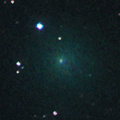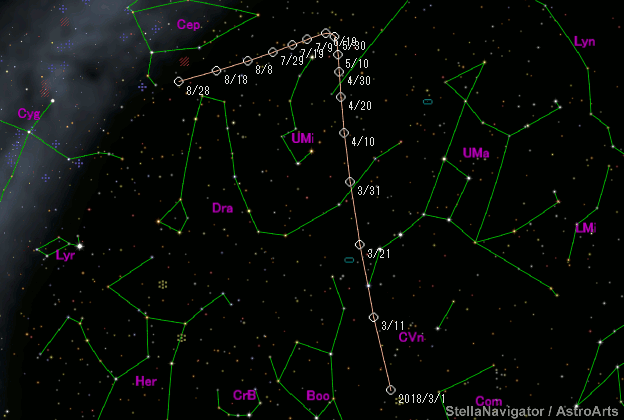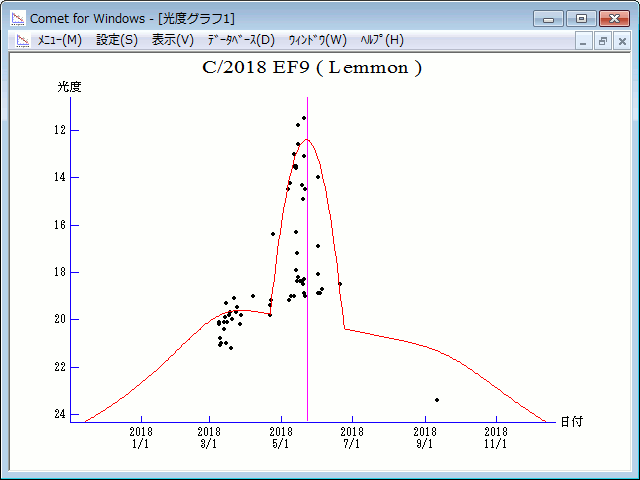# \$B%l%b%sWB@1(B

C/2018 EF9 ( Lemmon )###\$B%W%m%U%#!<%k(B

 \$BH/8+F|(B 2018\$BG/(B3\$B7n(B9\$BF|(B \$BH/8+8wEY(B 20.7\$BEy(B \$BH/8+ A. R. Gibbs (Mount Lemmon)

###\$B###\$B50F;MWAG(B

```Epoch 2018 June 11.0 TT = JDT 2458280.5
T 2018 May 23.13007 TT                                  MPCW
q   1.5566299            (2000.0)            P               Q
z  +0.0015592      Peri.   72.61780     -0.30764081     +0.94276858
+/-0.0000044      Node   172.57784     -0.42286504     -0.25656446
e   0.9975729      Incl.   84.69430     +0.85237450     +0.21298378
From 170 observations 2018 Mar. 9-Sept. 11, mean residual 0".6.
```

###\$B@1?^(B###\$B8wEYJQ2=(B

```        m1 =  17.0 + 5 log\$B&\$(B +  10.0 log r  [   ,-31]  (              \$B!A(B2018\$BG/(B 4\$B7n(B22\$BF|(B)
m1 = -81   + 5 log\$B&\$(B + 480   log r  [-31, 31]  (2018\$BG/(B 4\$B7n(B22\$BF|!A(B2018\$BG/(B 6\$B7n(B23\$BF|(B)
m1 =  17.0 + 5 log\$B&\$(B +  10.0 log r  [ 31,   ]  (2018\$BG/(B 6\$B7n(B23\$BF|!A(B              )
```##### \$B50F;MWAG\$O(BM.P.E.C. 2018-S50\$B\$K7G:\\$5\$l\$?\$b\$N\$G\$9!#(B \$B8wEY%0%i%U\$O(BComet for Windows\$B\$G:n@.\$7\$?\$b\$N\$G\$9!#(B